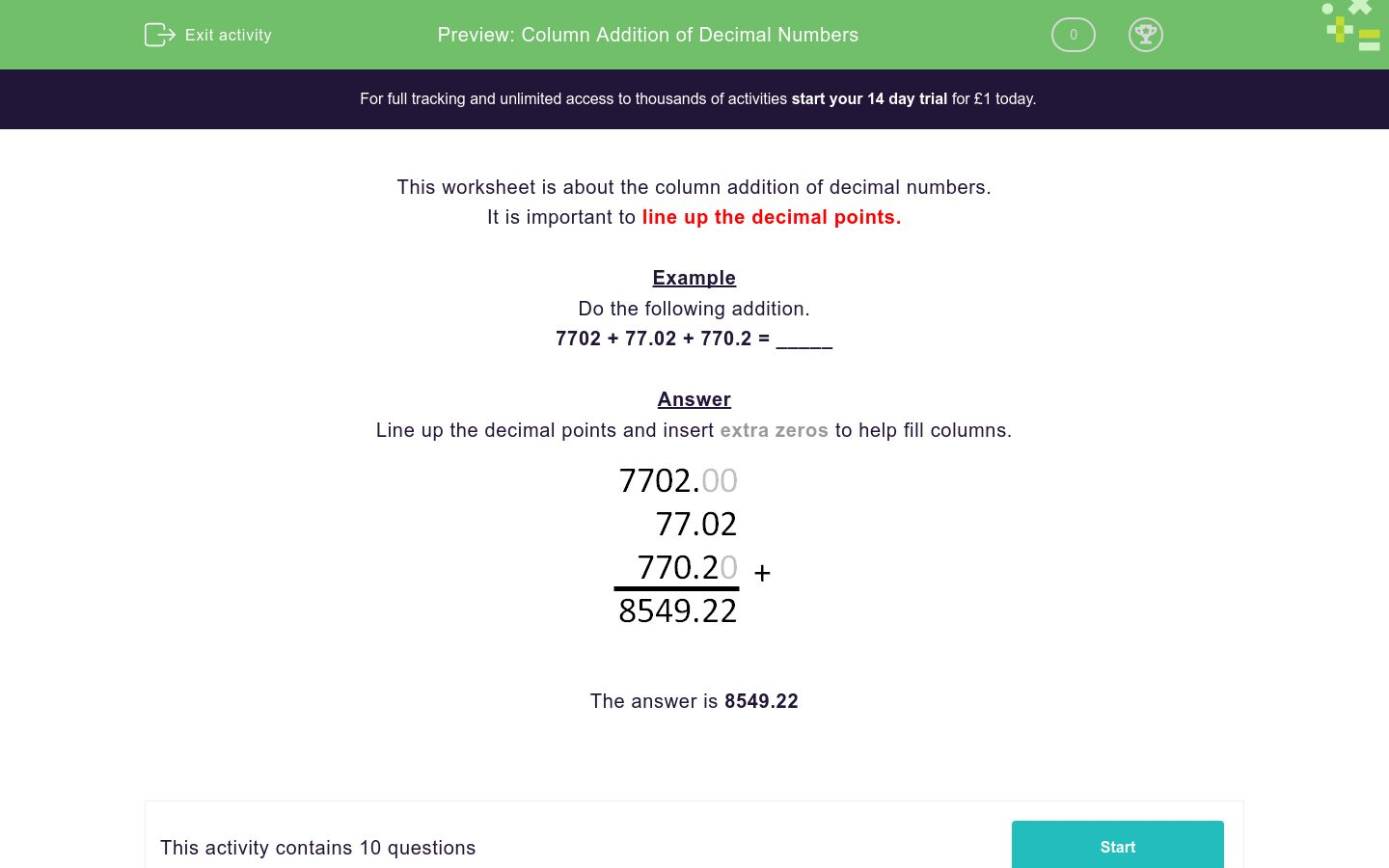# Column Addition of Decimal Numbers

In this worksheet, students practise adding decimal numbers using columns.Key stage:  KS 3

Curriculum topic:   Number

Curriculum subtopic:   Use Four Operations for All Numbers

Difficulty level:### QUESTION 1 of 10

It is important to line up the decimal points.

Example

7702 + 77.02 + 770.2 = _____

Line up the decimal points and insert extra zeros to help fill columns.596.1 + 5.961 + 59.61 = _____

3150.5 + 31.505 + 315.05 = _____

6284.7 + 62.847 + 628.47 = _____

1954.4 + 19.544 + 195.44 = _____

4956 + 49.56 + 495.6 = _____

840.3 + 8.403 + 84.03 = _____

7469.3 + 74.693 + 746.93 = _____

465.2 + 4.652 + 46.52 = _____

7561.9 + 75.619 + 756.19 = _____

1100 + 110 + 1.1 + 0.11 = _____

• Question 1

596.1 + 5.961 + 59.61 = _____

661.671
EDDIE SAYS

Line up the numbers using the decimal places.

Remember to add extra zeros on the end so each number has the same amount of decimal places.

 596.1 5.961 59.61
• Question 2

3150.5 + 31.505 + 315.05 = _____

3497.055
EDDIE SAYS

Line up the numbers using the decimal places.

Remember to add extra zeros on the end so each number has the same amount of decimal places.

 3150.5 31.505 315.05
• Question 3

6284.7 + 62.847 + 628.47 = _____

6976.017
EDDIE SAYS

Line up the numbers using the decimal places.

Remember to add extra zeros on the end so each number has the same amount of decimal places.

 6284.7 62.847 628.47
• Question 4

1954.4 + 19.544 + 195.44 = _____

2169.384
EDDIE SAYS

Line up the numbers using the decimal places.

Remember to add extra zeros on the end so each number has the same amount of decimal places.

 1954.4 19.544 195.44
• Question 5

4956 + 49.56 + 495.6 = _____

5501.16
EDDIE SAYS

Line up the numbers using the decimal places.

Remember to add extra zeros on the end so each number has the same amount of decimal places.

 4956 49.56 495.6
• Question 6

840.3 + 8.403 + 84.03 = _____

932.733
EDDIE SAYS

Line up the numbers using the decimal places.

Remember to add extra zeros on the end so each number has the same amount of decimal places.

 840.3 8.403 84.03
• Question 7

7469.3 + 74.693 + 746.93 = _____

8290.923
EDDIE SAYS

Line up the numbers using the decimal places.

Remember to add extra zeros on the end so each number has the same amount of decimal places.

 7469.3 74.693 746.93
• Question 8

465.2 + 4.652 + 46.52 = _____

516.372
EDDIE SAYS

Line up the numbers using the decimal places.

Remember to add extra zeros on the end so each number has the same amount of decimal places.

 465.2 4.652 46.52
• Question 9

7561.9 + 75.619 + 756.19 = _____

8393.709
EDDIE SAYS

Line up the numbers using the decimal places.

Remember to add extra zeros on the end so each number has the same amount of decimal places.

 7561.9 75.619 756.19
• Question 10

1100 + 110 + 1.1 + 0.11 = _____

1211.21
EDDIE SAYS

Line up the numbers using the decimal places.

Remember to add extra zeros on the end so each number has the same amount of decimal places.

 1100 110 1.1 0.11
---- OR ----